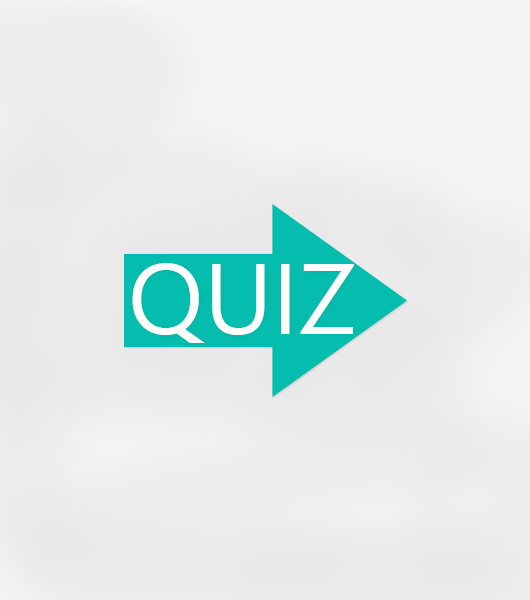# File Test 01 Vocabulary 4 complete The Maths. Tick The Correct Answer.

6 Questions | Attempts: 238
ShareSettings• 1.
Three + two =            A  five          B  six          C  seven
• A.

A

• B.

B

• C.

C

• 2.
Four + fifteen =     A  seventeen          B  nineteen          C  twenty
• A.

A

• B.

B

• C.

C

• 3.
Nine – seven =     A  one          B  two          C three
• A.

A

• B.

B

• C.

C

• 4.
Seven + eleven =     A  sixteen          B  seventeen          C  eighteen
• A.

A

• B.

B

• C.

C

• 5.
Nineteen – seven =     A  eleven          B  twelve          C  thirteen
• A.

A

• B.

B

• C.

C

• 6.
Thirteen + eighteen – twenty =     A  eleven          B  fifteen          C  twelve
• A.

A

• B.

B

• C.

C

## Related TopicsBack to top
×

Wait!
Here's an interesting quiz for you.